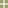Inference and Sampling DistributionsMain Concepts  | Demonstration  | Activity  | Teaching Tips  | Data Collection & Analysis  | Practice Questions  | Milestone   | Fathom TutorialTeaching Tips • Students must be able to distinguish between samples and populations and between statistics and parameters before they can begin to understand a sampling distribution. • From now on, you can't use the word "mean" alone. There is a mean for each sample, the mean of the population, and the mean of a sample statistic over many samples. • "Bias" is one of those terms that students have a hard time with because it has a different meaning in everyday English, where "bias" may mean "prejudice". In Statistics, it means that, on average, our estimate of a parameter is different from the true value of the parameter. So a butcher who puts his thumb on the scale introduces bias into his measurements, even if he only does this sometimes, because on average, he's adding weight to the scale. • When texts use the succinct equation µxbar = µ, they are referring to three different means: the mean of the population (µ), the mean of a sample (xbar), and the expected value of the collection of sample means (µxbar). This statement is another way of saying that the sample mean is an unbiased estimator of the population mean. (Incidentally, the equation µxbar = µ is valued for its succinctness rather than for its clarity.) •The standard error measures the "precision" or "error" of an estimate and is based on the spread of the population and the sample size. The larger the population standard deviation the larger the standard error and the larger the sample size the smaller the standard error. The standard error does not depend on the size of the population unless the population is relatively small with respect to the size of the sample. Students find this last feature to be very counter-intuitive. • The best way to teach sampling distributions is to simulate many samples. We suggest beginning with hands-on activities to help introduce the concept of the Central Limit Theorem rather than a technology-based simulation in which students passively observe. Example: ask students to come up and make a dot-plot of the last four digits of their phone numbers so that the entire class' digits are displayed on one graph. Before doing this, ask them what shape they think this distribution will be. Then ask them to find the average of their last four digits and plot this. The shape of the distribution of averages will be different from the shape of the distribution of single digits (which should be uniform-ish). Warning: the Central Limit Theorem says that the distribution of averages should be normal. But the sample size here is only 4, and so it might not look terribly normal. It will, however, look different from the distribution of single digits. • Students will confuse the size of the sample, n, with the number of simulated samples. (For example, in the telephone activity above, if you ask students what the sample size is, they'll often say that it's the number of students in the class. But this is the number of samples. The size of each sample is 4.) • Students will sometimes say, "I can't say anything until I've taken more samples," but the point of inference is to make a conclusion/decision based on just one sample. We might simulate multiple samples to help understand the nature of an estimator and its sampling distribution. But when we want to understand the population or answer a question about the world, then we get only one sample to do this. • In the probability simulation unit (Unit 8), we talked about using simulations to approximate probabilities of calculated probability problems. Here we're using simulations to help us understand properties of estimators. • Simulating more samples makes the picture of the sampling distribution come into clearer "focus", but it does not affect the shape of the sampling distribution. However, increasing the number of observations in each sample does change the shape of the sampling distribution. So if you have a skewed population distribution, the distribution of the means of small samples will also be skewed. But the distribution of means of large samples will be approximately normal. • The textbooks tend to have a narrow focus and concentrate only on means and proportions. But the concepts we're talking about here apply to any statistic and, in fact, you can test your own understanding of these concepts by seeing how well they work on unusual statistics. In this unit's Milestone we'll give you some practice with "unorthodox" statistics. • Why does the Central Limit Theorem apply to both sample proportions and sample means? Because sample proportions are sample means if your data consist of only 1's and 0's. If your data are 1,0,0,1,1,1, for example, than the average of these 6 numbers is the same thing as the proportion of 1's. This might not be something you need to discuss with your students, who might be more comfortable keeping proportions and means separate in their minds. • The INSPIRE workshop held in the summer of 2004 introduced more examples of simimulations to learn the CLT.  Check out the Reese's Pieces simulation, for example.